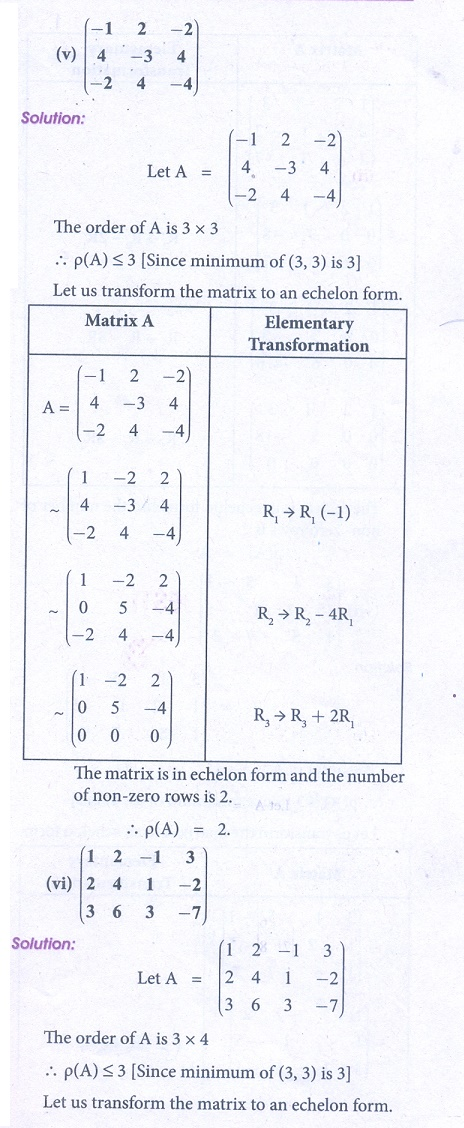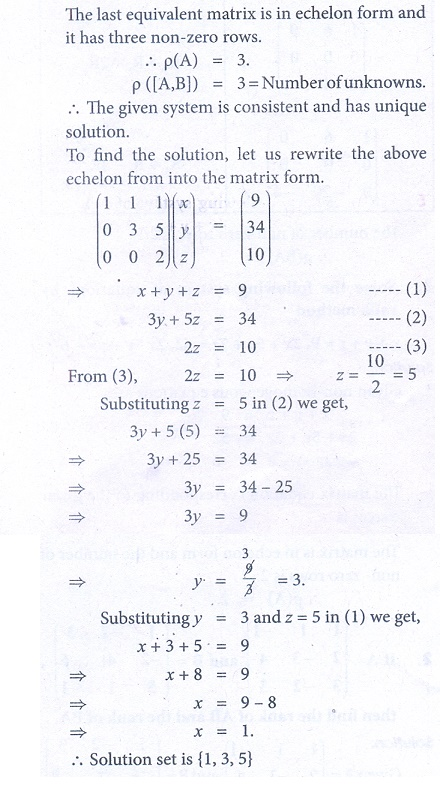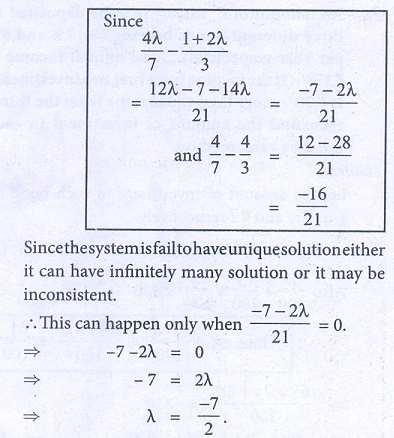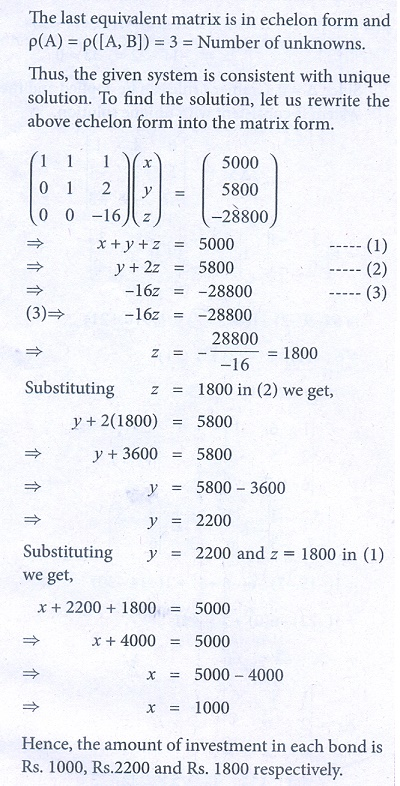Home | | Business Maths 12th Std | Exercise 1.1 : Rank of a Matrix

# Exercise 1.1 : Rank of a Matrix

Book back answers and solution for Exercise questions - Business Maths and Statistics : Applications of Matrices and Determinants: Solved Example Problems

Exercise 1.1

1. Find the rank of each of the following matrices2. If A =and B =, then find the rank of AB and the rank of BA.3. Solve the following system of equations by rank method

+ y + z = 9, 2+ 5+ 7= 52, 2x ŌłÆ y ŌłÆ z = 04. Show that the equations 5x + 3y + 7z = 4, 3x + 26 y + 2z = 9, 7x + 2 y + 10z = 5 are consistent and solve them by rank method.5. Show that the following system of equations have unique solution:

+ y + z = 3, x + 2 y + 3= 4, x + 4 y + 9= 6 by rank method.6. For what values of the parameter ╬╗ , will the following equations fail to have unique solution: 3x ŌłÆ y + ╬╗ z = 1, 2x + y + z = 2, x + 2 y ŌłÆ ╬╗ z = ŌłÆ1 by rank method.7. The price of three commodities X,Y and Z are x,y and z respectively Mr.Anand purchases 6 units of Z and sells 2 units of X and 3 units of Y. Mr.Amar purchases a unit of Y and sells 3 units of X and 2units of Z. Mr.Amit purchases a unit of X and sells 3 units of Y and a unit of Z. In the process they earn Ōé╣5,000/-, Ōé╣2,000/- and Ōé╣5,500/- respectively Find the prices per unit of three commodities by rank method.8. An amount of Ōé╣5,000/- is to be deposited in three different bonds bearing 6%, 7% and 8% per year respectively. Total annual income is Ōé╣358/-. If the income from first two investments is Ōé╣70/- more than the income from the third, then find the amount of investment in each bond by rank method.